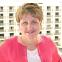Java Games: Flashcards, matching, concentration, and word search.# Browns Equations

Math 7

AB
x + 7 = -2x = -9
2x = 8x = 4
-2 + x = -10x = -8
10 / x = 2x = 5
2x + 1 = 3x = 1
14 / x + 2 = 2x = 0
-5 + x = -7x = -2
-20x = -40x = 2
1 + x = 11x = 10
-1 + x = -12x = -11
-1 + x = 12x = 13
6x =36x = 6Mrs. Cardwell FACS Instructor Shawnee Heights Tecumseh, KS

 This activity was created by a Quia Web subscriber. Learn more about QuiaCreate your own activities